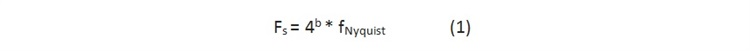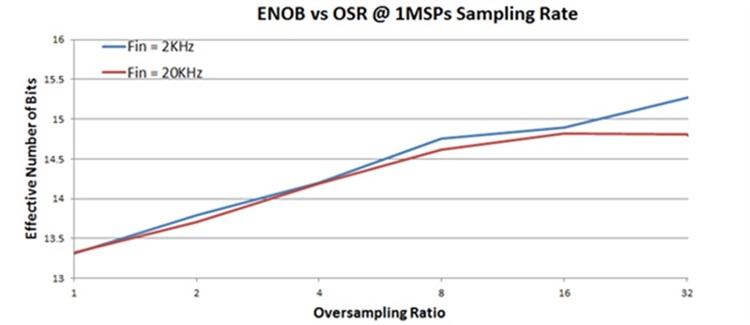Other Parts Discussed in Post: MSP432P401R

Have you ever found an analog-to-digital converter (ADC) for your application and later realized that you needed higher effective resolution? If you have noise in your system or can add noise to your ADC input signal, you may be in luck. Oversampling the signal can increase the effective number of bits (ENOB) of resolution for the ADC, but this requires the presence of noise. In some cases, however, you can intentionally add noise to the signal to enable oversampling.

Oversampling means to sample at higher than the Nyquist rate, which is two times the signal’s maximum frequency component. Averaging, or a low-pass filter followed by decimation typically follows oversampling. Oversampling can result in increased effective ADC resolution. For each bit of additional resolution desired, you must oversample by a factor of four. See Equation 1:Where b is the number of extra effective bits, FNyquist is the nyquist frequency.

The key is knowing when you can oversample.

Some ADCs have a built-in hardware oversampling mode that averages the converted output; see Figure 1, option A. On a microcontroller (MCU) with an integrated ADC, you can also leverage software to implement oversampling (see the application note ‘General Oversampling of MSP ADCs for Higher Resolution’ for more details and a link to access code example projects). In software oversampling, the MCU performs post-processing (including post-averaging), or uses a low-pass filter and decimation. The low-pass filter takes more processing bandwidth (and power) but removes out-of-band noise for better performance.Figure 1: Oversampling method signal-flow diagram

Take the MSP432P401R MCU as an example: This microcontroller includes an integrated 1 MSPS, Precision ADC. To increase the ADC’s 13.2 ENOB of resolution to 14.2 bits for a 20 kHz signal, it must be sampled at a minimum of four times the Nyquist rate (40 kHz), which is 160 kHz. To go from 13.2 to 15.2 bits, it must be sampled at a minimum of 42 = 16 times the Nyquist rate, which is 640 kHz (for sigma delta ADCs, the 60 kHz is not necessarily the same as the clock rate).

Figure 2 shows the performance of the MSP432P401R MCU’s Precision ADC with oversampling. Note that when the input signal’s frequency is 20 kHz, performance stops improving at an oversampling rate (OSR) of 32. That is because the maximum sample frequency of the ADC is 1 Msps, and because the converter is 1 MSPS, the maximum the signal can be at an OSR of 32 to increase the ENOB is 15.625 kHz (based on Equation 1).Figure 2: MSP432P401R MCU ADC performance with different oversampling ratios

Going back to “the key is knowing when you can oversample,” this is where noise is the secret to increasing your ADC’s effective resolution. Here are a few high-level requirements for oversampling to be effective at increasing resolutions:

• Noise on the system should be large enough to create a difference in successive ADC conversion. This noise can be input noise or noise inside the converter (quantization plus thermal).
• Noise should be white if you are not using decimation filters.
• Band-limited non-stationary signals benefit more from oversampling.

If the signal does not have enough noise, you can add out-of-band noise to get better performance through oversampling, and then filter out the added noise.

Keep in mind these points about oversampling:

• The converter sample rate must be greater than two times the maximum signal frequency.
• Oversampling increases power, so only use it if necessary – and only to the degree necessary.
• Oversampling is not as simple as having an ADC that has the required ENOB of resolution where the results are directly outputted. Oversampling requires additional processing after the ADC result.
• You will need additional post-processing in software. Pre-verified software combined with a high-performance central processing unit (CPU) such as the MSP432™ MCU can help you quickly implement your oversampling ADC solution.